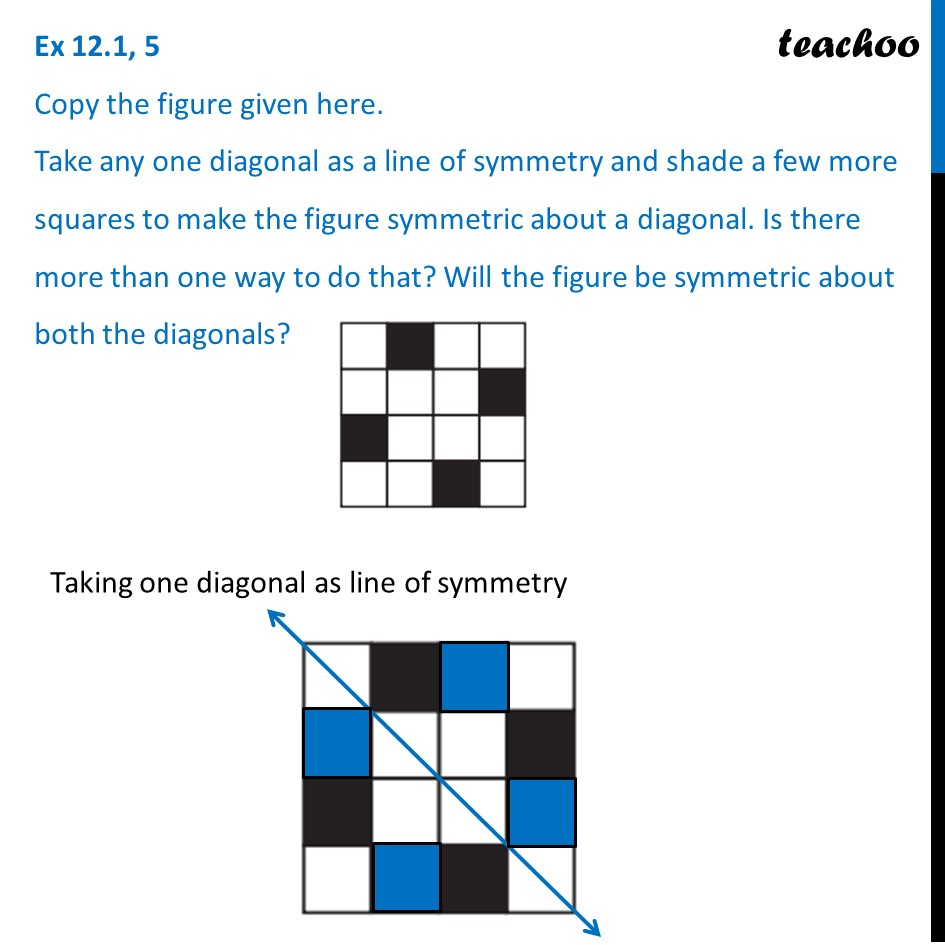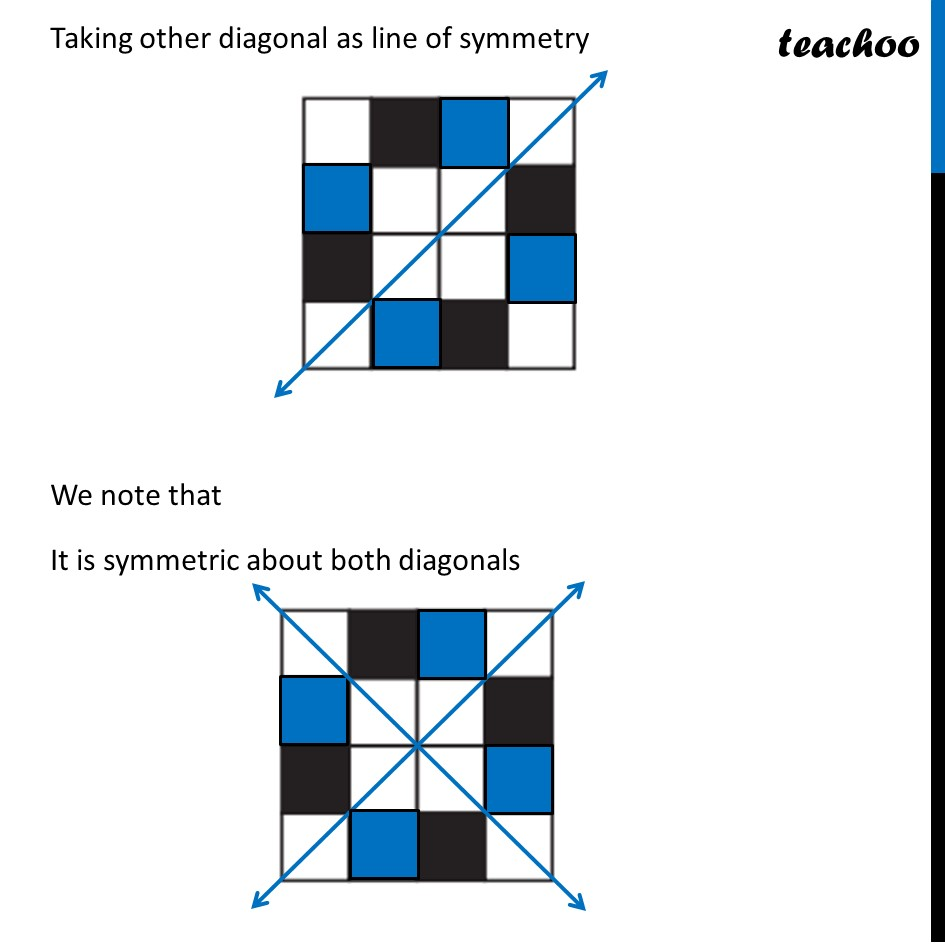Ex 12.1

Chapter 12 Class 7 Symmetry
Serial order wiseLearn in your speed, with individual attention - Teachoo Maths 1-on-1 Class

### Transcript

Ex 12.1, 5 Copy the figure given here. Take any one diagonal as a line of symmetry and shade a few more squares to make the figure symmetric about a diagonal. Is there more than one way to do that? Will the figure be symmetric about both the diagonals? Taking one diagonal as line of symmetry Taking other diagonal as line of symmetry We note that It is symmetric about both diagonals